Next: Gaussian variable with nonlinearity Up: Building Blocks Previous: Gaussian variables

## Addition and Multiplication

Addition and multiplication nodes can be used e.g. for constructing linear mappings and affine transformations between the variables. Denoting the inputs by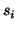, the outputs are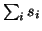for addition and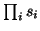for multiplication nodes. The mean, variance and expected exponential of the addition node are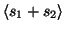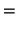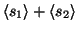(3)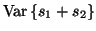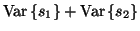(4)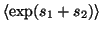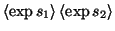(5)

assumingindependent. For a multiplication node, the expected exponential cannot be evaluated without knowing the exact distribution of the inputs. Assuming independence between, the mean and the variance of the output are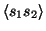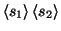(6)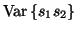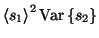(7)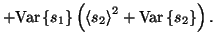The equations for larger sums or products are obtained by induction, e.g.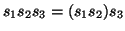.Next: Gaussian variable with nonlinearity Up: Building Blocks Previous: Gaussian variables
Harri Valpola 2001-10-01